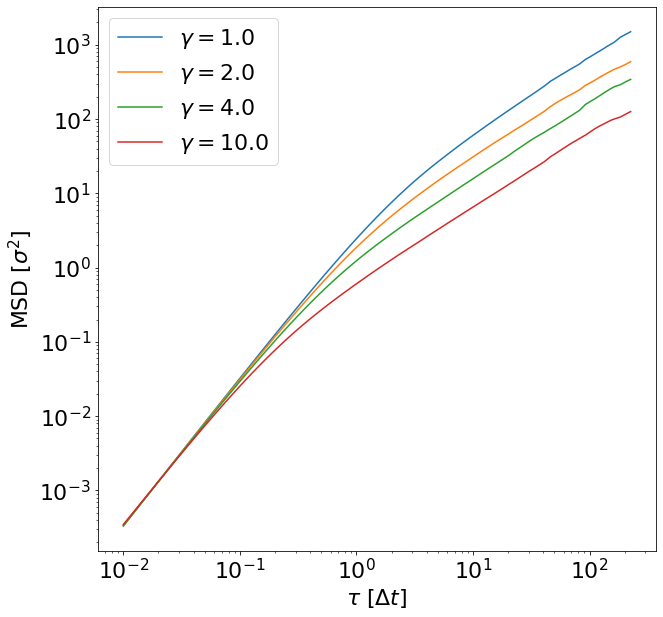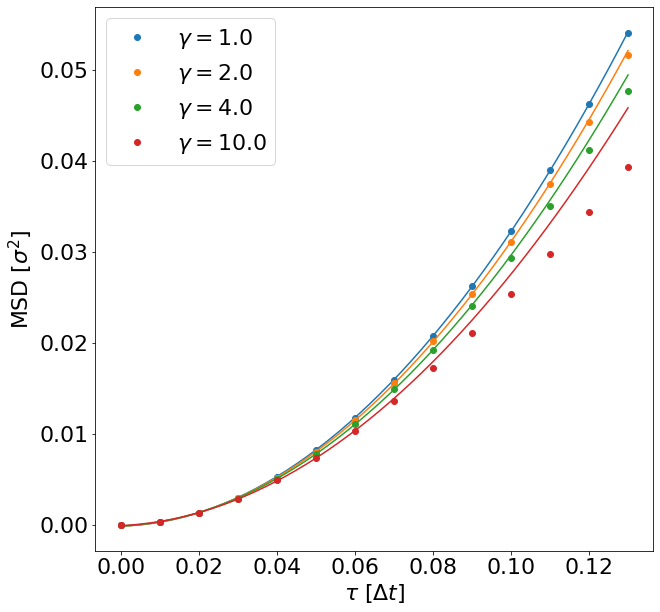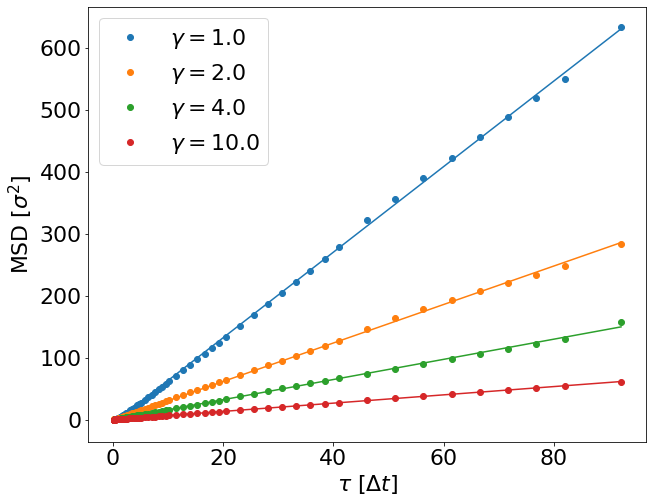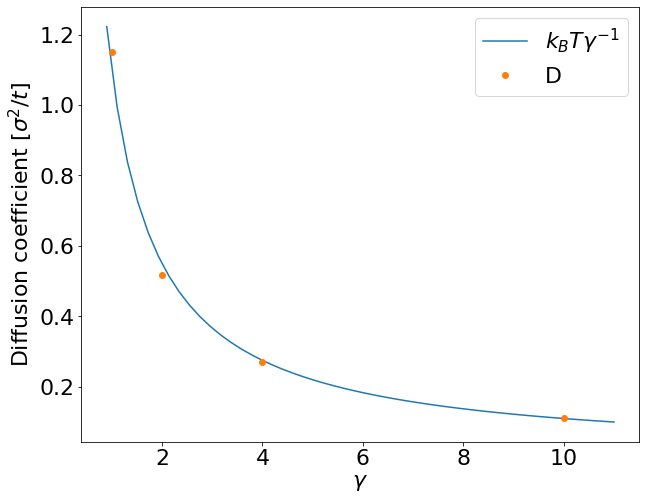# Tutorial 4 : The lattice-Boltzmann method in ESPResSo - Part 2¶

## Diffusion of a single particle¶

In these exercises we want to reproduce a classic result of polymer physics: the dependence of the diffusion coefficient of a polymer on its chain length. If no hydrodynamic interactions are present, one expects a scaling law $D \propto N ^{- 1}$ and if they are present, a scaling law $D \propto N^{- \nu}$ is expected. Here $\nu$ is the Flory exponent that plays a very prominent role in polymer physics. It has a value of $\sim 3/5$ in good solvent conditions in 3D. Discussions on these scaling laws can be found in polymer physics textbooks like [4–6].

The reason for the different scaling law is the following: when being transported, every monomer creates a flow field that follows the direction of its motion. This flow field makes it easier for other monomers to follow its motion. This makes a polymer (given it is sufficiently long) diffuse more like a compact object including the fluid inside it, although it does not have clear boundaries. It can be shown that its motion can be described by its hydrodynamic radius. It is defined as:

\begin{equation} \left\langle \frac{1}{R_h} \right\rangle = \left\langle \frac{1}{N^2}\sum_{i\neq j} \frac{1}{\left| r_i - r_j \right|} \right\rangle \end{equation}

This hydrodynamic radius exhibits the scaling law $R_h \propto N^{\nu}$ and the diffusion coefficient of a long polymer is proportional to its inverse $R_h$.

For shorter polymers there is a transition region. It can be described by the Kirkwood–Zimm model:

\begin{equation} D=\frac{D_0}{N} + \frac{k_B T}{6 \pi \eta } \left\langle \frac{1}{R_h} \right\rangle \end{equation}

Here $D_0$ is the monomer diffusion coefficient and $\eta$ the viscosity of the fluid. For a finite system size the second part of the diffusion is subject to a $1/L$ finite size effect, because hydrodynamic interactions are proportional to the inverse distance and thus long ranged. It can be taken into account by a correction:

\begin{equation} D=\frac{D_0}{N} + \frac{k_B T}{6 \pi \eta } \left\langle \frac{1}{R_h} \right\rangle \left( 1- \left\langle\frac{R_h}{L} \right\rangle \right) \end{equation}

It is quite difficult to prove this formula computationally with good accuracy. It will need quite some computational effort and a careful analysis. So please don't be too disappointed if you don't manage to do so.

We want to determine the long-time self diffusion coefficient from the mean square displacement of the center-of-mass of a single polymer. For large $t$ the mean square displacement is proportional to the time and the diffusion coefficient occurs as a prefactor:

\begin{equation} D = \lim_{t\to\infty}\left[ \frac{1}{6t} \left\langle \left(\vec{r}(t) - \vec{r}(0)\right)^2 \right\rangle \right]. \end{equation}

This equation can be found in virtually any simulation textbook, like . We will set up a polymer in an implicit solvent, simulate for an appropriate amount of time, calculate the mean square displacement as a function of time and obtain the diffusion coefficient from a linear fit. However we will have a couple of steps inbetween and divide the full problem into subproblems that allow to (hopefully) fully understand the process.

### 1. Setting up the observable¶

Write a function with signature correlator_msd(pid, tau_max) that returns a mean-squared displacement correlator that is updated every time step.

In :
def correlator_msd(pid, tau_max):
pos = espressomd.observables.ParticlePositions(ids=(pid,))
pos_cor = espressomd.accumulators.Correlator(
obs1=pos, tau_lin=16, tau_max=tau_max, delta_N=1,
return pos_cor


### 2. Simulating the Brownian motion¶

We will simulate the diffusion of a single particle that is coupled to an implicit solvent.

In :
import numpy as np
import logging
import sys

import espressomd
import espressomd.accumulators
import espressomd.observables

logging.basicConfig(level=logging.INFO, stream=sys.stdout)

# Constants
KT = 1.1
STEPS = 400000

# System setup
system = espressomd.System(box_l= * 3)
system.time_step = 0.01
system.cell_system.skin = 0.4

# Run for different friction coefficients
gammas = [1.0, 2.0, 4.0, 10.0]
tau_results = []
msd_results = []

for gamma in gammas:
system.auto_update_accumulators.clear()
system.thermostat.turn_off()
system.thermostat.set_langevin(kT=KT, gamma=gamma, seed=42)

logging.info("Equilibrating the system.")
system.integrator.run(1000)
logging.info("Equilibration finished.")

# Setup observable correlator
correlator = correlator_msd(0, STEPS)

logging.info("Sampling started for gamma = {}.".format(gamma))
system.integrator.run(STEPS)
correlator.finalize()
tau_results.append(correlator.lag_times())
msd_results.append(np.sum(correlator.result().reshape([-1, 3]), axis=1))

logging.info("Sampling finished.")

INFO:root:Equilibrating the system.
INFO:root:Equilibration finished.
INFO:root:Sampling started for gamma = 1.0.
INFO:root:Equilibrating the system.
INFO:root:Equilibration finished.
INFO:root:Sampling started for gamma = 2.0.
INFO:root:Equilibrating the system.
INFO:root:Equilibration finished.
INFO:root:Sampling started for gamma = 4.0.
INFO:root:Equilibrating the system.
INFO:root:Equilibration finished.
INFO:root:Sampling started for gamma = 10.0.
INFO:root:Sampling finished.


### 3. Data analysis¶

#### 3.1 Plotting the results¶

In :
%matplotlib inline
import matplotlib.pyplot as plt

In :
plt.rcParams.update({'font.size': 22})

plt.figure(figsize=(10, 10))
plt.xlabel(r'$\tau$ [$\Delta t$]')
plt.ylabel(r'MSD [$\sigma^2$]')
for index, (tau, msd) in enumerate(zip(tau_results, msd_results)):
# We skip the first entry since it's zero by definition and cannot be displayed
# in a loglog plot. Furthermore, we only look at the first 100 entries due to
# the high variance for larger lag times.
plt.loglog(tau[1:100], msd[1:100], label=r'$\gamma=${:.1f}'.format(gammas[index]))
plt.legend()
plt.show()#### 3.2 Calculating the diffusion coefficient¶

In this script an implicit solvent and a single particle are created and thermalized. The random forces on the particle will cause the particle to move. The mean squared displacement is calculated during the simulation via a multiple-tau correlator. Can you give an explanation for the quadratic time dependency for short times?

The MSD of a Brownian motion can be decomposed in three main regimes :

• for short lag times $\tau < \tau_p$, the particle motion is not significantly impeded by solvent collisions: it's in the ballistic mode (collision-free regime) where $\operatorname{MSD}(t) \sim (k_BT / m) t^2$
• for long lag times $\tau > \tau_f$, the particle motion is determined by numerous collisions with the solvent: it's in the diffusive mode where $\operatorname{MSD}(t) \sim 6t$
• for lag times between $\tau_p$ and $\tau_f$, there is a crossover mode

The values $\tau_p$ and $\tau_f$ can be obtained manually through visual inspection of the MSD plot, or more accurately by non-linear fitting .

The cutoff lag time $\tau_p$ between the ballistic and crossover modes is proportional to the particle mass and inversely proportional to the friction coefficient. In the graph below, a parabola is fitted to the data points in the ballistic mode for each $\gamma$ and plotted beyond the crossover region to reveal the deviation from the ballistic mode. This deviation is clearly visible in the $\gamma = 10$ case, because the assumption of a collision-free regime quickly breaks down when a particle is coupled to its surrounding fluid with a high friction coefficient.

In :
import scipy.optimize

return a * x**2 + b * x + c

# cutoffs for the ballistic regime (different for each gamma value)
tau_p_values = [14, 12, 10, 7]

plt.figure(figsize=(10, 10))
plt.xlabel(r'$\tau$ [$\Delta t$]')
plt.ylabel(r'MSD [$\sigma^2$]')
for index, (tau_p, tau, msd) in enumerate(zip(tau_p_values, tau_results, msd_results)):
(a, b, c), _ = scipy.optimize.curve_fit(quadratic, tau[:tau_p], msd[:tau_p])
x = np.linspace(tau, tau[max(tau_p_values) - 1], 50)
p = plt.plot(x, quadratic(x, a, b, c), '-')
plt.plot(tau[:max(tau_p_values)], msd[:max(tau_p_values)], 'o', color=p.get_color(),
label=r'$\gamma=${:.1f}'.format(gammas[index]))
plt.legend()
plt.show()Use the function curve_fit() from the module scipy.optimize to produce a fit for the linear regime and determine the diffusion coefficients for the different $\gamma$s.

For large $t$ the diffusion coefficient can be expressed as:

$$6D = \lim_{t\to\infty} \frac{\partial \operatorname{MSD}(t)}{\partial t}$$

which is simply the slope of the MSD in the diffusive mode.

In :
def linear(x, a, b):
return a * x + b

# cutoffs for the diffusive regime (different for each gamma value)
tau_f_values = [24, 22, 20, 17]
# cutoff for the data series (larger lag times have larger variance due to undersampling)
cutoff_limit = 90

diffusion_results = []

plt.figure(figsize=(10, 8))
plt.xlabel(r'$\tau$ [$\Delta t$]')
plt.ylabel(r'MSD [$\sigma^2$]')
for index, (tau_f, tau, msd) in enumerate(zip(tau_f_values, tau_results, msd_results)):
(a, b), _ = scipy.optimize.curve_fit(linear, tau[tau_f:cutoff_limit], msd[tau_f:cutoff_limit])
x = np.linspace(tau[tau_f], tau[cutoff_limit - 1], 50)
p = plt.plot(x, linear(x, a, b), '-')
plt.plot(tau[tau_f:cutoff_limit], msd[tau_f:cutoff_limit], 'o', color=p.get_color(),
label=r'$\gamma=${:.1f}'.format(gammas[index]))
diffusion_results.append(a / 6)

plt.legend()
plt.show()Calculate the diffusion coefficient for all cases and plot them as a function of $\gamma$. What relation do you observe?

In the diffusive mode, one can derive $D = k_BT / \gamma$ from the Stokes–Einstein relation .

In :
plt.figure(figsize=(10, 8))
plt.xlabel(r'$\gamma$')
plt.ylabel('Diffusion coefficient [$\sigma^2/t$]')
x = np.linspace(0.9 * min(gammas), 1.1 * max(gammas), 50)
y = KT / x
plt.plot(x, y, '-', label=r'$k_BT\gamma^{-1}$')
plt.plot(gammas, diffusion_results, 'o', label='D')
plt.legend()
plt.show()S. Succi. The lattice Boltzmann equation for fluid dynamics and beyond. Clarendon Press, Oxford, 2001.
 B. Dünweg and A. J. C. Ladd. Advanced Computer Simulation Approaches for Soft Matter Sciences III, chapter II, pages 89–166. Springer, 2009.
 B. Dünweg, U. Schiller, and A.J.C. Ladd. Statistical mechanics of the fluctuating lattice-Boltzmann equation. Phys. Rev. E, 76:36704, 2007.
 P. G. de Gennes. Scaling Concepts in Polymer Physics. Cornell University Press, Ithaca, NY, 1979.
 M. Doi. Introduction to Polymer Physics. Clarendon Press, Oxford, 1996.
 Michael Rubinstein and Ralph H. Colby. Polymer Physics. Oxford University Press, Oxford, UK, 2003.
 Daan Frenkel and Berend Smit. Understanding Molecular Simulation, section 4.4.1. Academic Press, San Diego, second edition, 2002.
 R. Huang, I. Chavez, K. Taute, et al. Direct observation of the full transition from ballistic to diffusive Brownian motion in a liquid. Nature Phys., 7, 2011. doi:10.1038/nphys1953
 M. K. Riahi, I. A. Qattan, J. Hassan, D. Homouz, Identifying short- and long-time modes of the mean-square displacement: An improved nonlinear fitting approach. AIP Advances, 9:055112, 2019. doi:10.1063/1.5098051